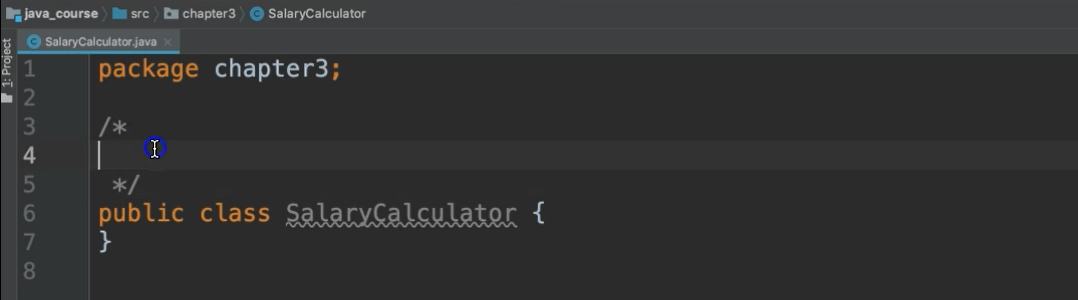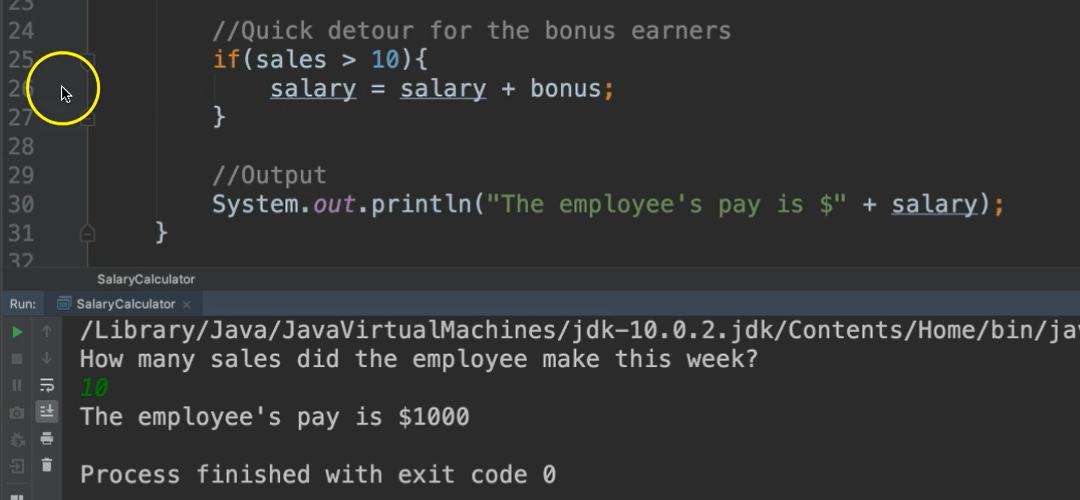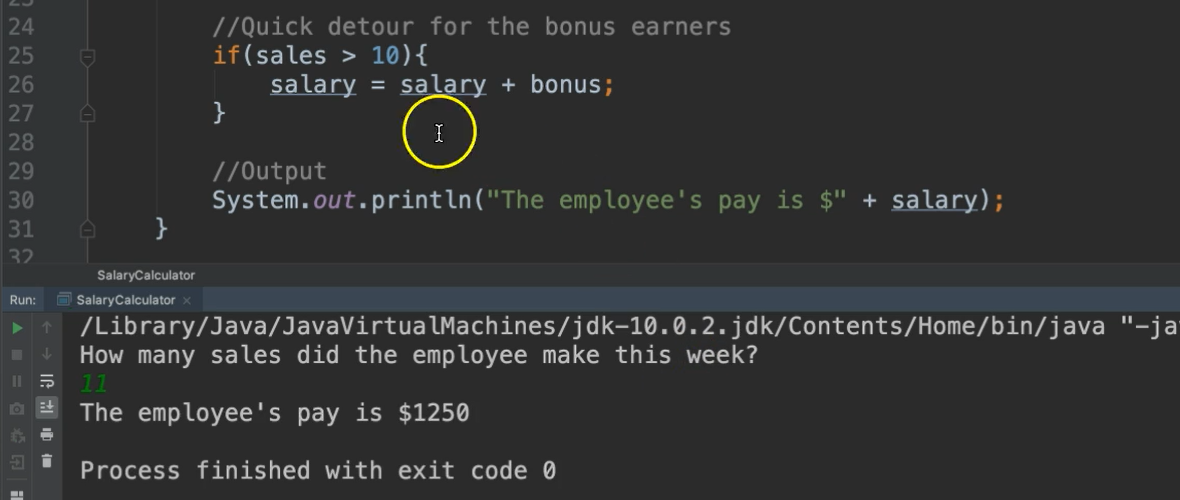Transcripted Summary

Oftentimes programs have to make decisions, so we need to provide different paths of execution within our code.

In this chapter, we'll look at various types of decision structures, as well as relational and logical operators, which help us evaluate conditions.

The `if` statement works as a quick detour within a program.

The If Statement

If a certain situation occurs, do something and then go back to the main flow.

If a certain situation occurs, the program does something and then goes back to the main flow of execution. Let's look at an example.Let's say we have a team of salespeople, all of whom make a standard rate of \$1,000 a week. For any of them who make more than 10 sales that week, they get an additional bonus of \$250.

So, the main path would be to pay a salesperson \$1,000 - everyone gets this no matter what. However, at some point we need to check to see if the condition was met of them making more than 10 sales. If it's met, then we need to add more to their payment.

Let's code this.

Let's make a new package.

We'll go back under this src folder and since we're doing stuff for chapter 3, we'll make a New Package: `chapter3`

Notice it's parallel with `chapter2`, so it's a totally different package.

Now we're going to create a new class.

We'll call this class `SalaryCalculator`.

I’m going to show you a new type of comment now. The ones that we've seen before have been like this (`//comment`) with the double slash. However, what if you wanted to have something that spans multiple lines? You don't want to keep typing the slashes for every line as it gets redundant.

I can use a different notation for a multi-line comment.

It's a slash followed by an asterisk: `/*`

And then notice as soon as I press enter it completed this comment with another asterisk and then a slash: `*/`So, anything that I type inside of here, no matter how many lines will be commented out.

Comments can go anywhere. I'm putting it in right here above the class to show you that it doesn't always have to be inside the class. It literally can be anywhere within this file, even at the top, as long as the first readable line of your class is the package.

So, we'll say:

``````/*
IF Statement.
All salespeople get a payment of \$1000 a week.
Salespeople who exceed 10 sales get an additional bonus of \$250.
*/
public class SalaryCalculator {

}
``````

Great.

So again, first thing we need is our main method.

``````public class SalaryCalculator {

public static void main(String args[]){

}
}
``````

Now what I'm going to do is I'm going to write the steps and comments of what I want to do, just thinking it through. So, really important, before you start coding that you think through the design of what it is that you're coding.

The first thing that I want to do is to initialize the known values. So, what do I already know?

`````` //Initialize known values
``````

And then get what I don't know.

``````//Get values for the unknown
``````

After I have all of the values that I need, then I can use my quick detour for the bonus earners.

``````//Quick detour for the bonus earners
``````

This will be when I make my decisions.

And then once I've done all of that, that's the calculation part, I'll do the output part.

``````//Output
``````

So again, figure out what I already know, get what I don't know, do my calculations, output my results. This gives us a nice path and a flow of what we want to do.

``````public static void main(String args[]){

//Initialize known values

//Get values for the unknown

//Quick detour for the bonus earners

//Output
}
``````

As far as initializing what we already know... in the last chapter, we didn't know anything, so, we had to receive all of our data from input. This time we have a little bit of information. We know that their salary is \$1,000 and we also know that the bonus is \$250.

So, I'm going to go ahead and declare these variables and then set their values. Giving them their initial values is called initializing.

``````    //Initialize known values
int salary = 1000;
int bonus = 250;
int quota = 10;
``````

What I don't know is how many sales did the employee make this week?

``````//Get values for the unknown
System.out.println("How many sales did the employee make this week?");
``````

And then we want to receive this input.

So, we use the Scanner:

``````Scanner scanner = new Scanner(System.in);
``````

Again, we need to import this. Notice that now the import statement is at the top:

``````import java.util.Scanner;

/*
IF Statement.
All salespeople get a payment of \$1000 a week.
Salespeople who exceed 10 sales get an additional bonus of \$250.
*/
public class SalaryCalculator {
``````

Let's go ahead and read the data in and we're going to store that in a variable as well.

``````int sales = scanner.nextInt();
``````

We're done getting our input, so let’s close the Scanner

``````scanner.close();
``````

Here's what we have so far

``````package chapter3;

import java.util.Scanner;

/*
IF Statement.
All salespeople get a payment of \$1000 a week.
Salespeople who exceed 10 sales get an additional bonus of \$250.
*/
public class SalaryCalculator {

public static void main(String args[]){

//Initialize known values
int salary = 1000;
int bonus = 250;
int quota = 10;

//Get values for the unknown
System.out.println("How many sales did the employee make this week?");
Scanner scanner = new Scanner(System.in);
int sales = scanner.nextInt();
scanner.close();

//Quick detour for the bonus earners

//Output
}

}
``````

Now, let's go to the next step.

So, a quick detour for the bonus earner. It says that if it a salesperson exceeds 10 sales, they get an additional bonus. So how do we know if it exceeds 10 sales? Well, we have to use that if statement.

So, the way you do an if statement is you write the word, `if` — this is a reserved word.

You follow `if` with parenthesis (`()`).

``````if()
``````

Inside of the parentheses is the condition that you need to evaluate. This condition must resolve to a boolean value.

::tip What is a Boolean value in Java? Boolean is another data type. Boolean values will be either true or false. That's the only type of data it holds: true or false. True or false can also be represented by the numbers 1 and 0 where 1 is true and 0 is false. ::

We use an expression inside of here that is a condition that which evaluate to true or false.

So, our expression will be: `sales > 10`

And this will evaluate to either true or false.

``````if(sales > 10)
``````

Once that's evaluated, we have our boolean value and then we have our braces for the `if`:

``````//Quick detour for the bonus earners
if(sales > 10){

}
``````

So, everything inside of these braces will be executed if the condition (`sales > 10`) results to true. If this does not result to true, then it will not go inside of these braces and it will continue on with the program.

So, let's put what needs to happen inside of the braces.

``````if(sales > 10){
salary = salary + bonus;
}
``````

We want to add the bonus to their salary. So, what we're doing here is essentially updating the salary variable. We say `salary =` and then we need to give it the value that it should be updated to. Well it depends on what's already in `salary`. So, we'll say `salary` again, then `+` for addition and then `bonus`:

``````salary = salary + bonus;
``````

What this is saying is to take this salary memory location which is represented on the left side of the equal sign and update it with the value which is expressed on the right side of the equal sign. So, take this memory location `salary` and update it with the value that's in that `salary` memory location already; add to that the value that's in the `bonus` memory location.

So, this variable `salary` now becomes the result of this sum.

Notice we did not say `int salary` this time because salary was already declared on line 15. We've already told our program the data type for this variable. We don't have to re-declare that every time we use it. This is only an update.

All right, now we have our calculation, we can go ahead and print this out.

``````//Output
System.out.println("The employee's pay is \$" + salary);
``````

Now notice here we use `+` two times for two different things. When this operator is used for numbers, it’s doing addition. When it's used with a String is doing appending.

All right? Let's run it.

### # SalaryCalculator.java

``````package chapter3;

import java.util.Scanner;

/*
IF Statement.
All salespeople get a payment of \$1000 a week.
Salespeople who exceed 10 sales get an additional bonus of \$250.
*/
public class SalaryCalculator {

public static void main(String args[]){

//Initialize known values
int salary = 1000;
int bonus = 250;

//Get values for the unknown
System.out.println("How many sales did the employee make this week?");
Scanner scanner = new Scanner(System.in);
int sales = scanner.nextInt();
scanner.close();

//Quick detour for the bonus earners
if(sales > quota){
salary = salary + bonus;
}

//Output
System.out.println("The employee's pay is \$" + salary);

}
}
``````

So how many sales did you make this week? Let's say we made the 10 sales that we required, so our pay is \$1000. Let's look at what happened.It came here and it said “if sales is greater than 10”, so sales held the number 10 in it. So, “is 10 greater than 10” is false. So, it never came inside of the if statement. And then it printed out the \$1000 so that's right.

Let's run it again using a different number this time. Let's say we didn't make our quota, we made 8 so it came to the if statement and asked “is 8 greater than 10”? No. So, we print out the \$1000.

Let’s run it again this time let's do greater than 10. This time “is 11 greater than 10”? Yes, true. So, it goes inside of the if statement and adds the \$250 to the \$1000 and then continued on with the program.Okay. There's one more thing here that I want you to be aware of.

In our condition we said `if(sales > 10)` and this is fine, and it makes sense to us for right now. However, if we revisit this program later on, then we would have no idea what this “10” means.

Why are they looking at the 10? What does this mean?

Magic Numbers

This is what's called a magic number in programming. It means that this number is just kind of out here and we have no idea what it means without doing some further research. I would have to scroll and come all the way up to the comments at the top of the program to see what this meant.

So instead of using the magic number, we can assign it to a variable and use the variable names so that it makes sense within context.

``````int quota = 10;
``````

Then we'll change this to use that variable:

``````if(sales > quota)
``````

And that gets rid of the magic number, which was hard coded before. We say hard coded when we are just putting the raw data as opposed to using the variable name.

``````        //Initialize known values
int salary = 1000;
int bonus = 250;
int quota = 10;

//Get values for the unknown
System.out.println("How many sales did the employee make this week?");
Scanner scanner = new Scanner(System.in);
int sales = scanner.nextInt();
scanner.close();

//Quick detour for the bonus earners
if(sales > quota){
salary = salary + bonus;
}

``````

So, there you go. That’s the ‘if` statement.